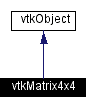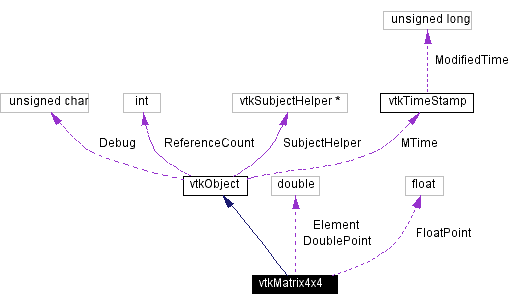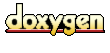Main Page   Class Hierarchy   Alphabetical List   Compound List   File List   Compound Members   File Members   Related Pages

# vtkMatrix4x4 Class Reference

represent and manipulate 4x4 transformation matrices. More...

`#include <vtkMatrix4x4.h>`

Inheritance diagram for vtkMatrix4x4:[legend]
Collaboration diagram for vtkMatrix4x4:[legend]
List of all members.

void Invert ()
void Invert (vtkMatrix4x4 *in, vtkMatrix4x4 *out)

void Transpose ()
void Transpose (vtkMatrix4x4 *in, vtkMatrix4x4 *out)

## Public Methods

virtual const char * GetClassName ()
virtual int IsA (const char *type)
void PrintSelf (ostream &os, vtkIndent indent)
double Determinant ()
void SetElement (int i, int j, double value)
double * operator[] (const unsigned int i)
const double * operator[] (unsigned int i) const
void operator= (double element)
void Adjoint (vtkMatrix4x4 &in, vtkMatrix4x4 &out)
double Determinant (vtkMatrix4x4 &in)
double Determinant (vtkMatrix4x4 *in)
void Invert (vtkMatrix4x4 &in, vtkMatrix4x4 &out)
void Transpose (vtkMatrix4x4 &in, vtkMatrix4x4 &out)
void DeepCopy (vtkMatrix4x4 *source)
void DeepCopy (const double Elements)
void Zero ()
void Identity ()
void MultiplyPoint (const float in, float out)
void MultiplyPoint (const double in, double out)
float * MultiplyPoint (const float in)
float * MultiplyFloatPoint (const float in)
double * MultiplyDoublePoint (const double in)
void Adjoint (vtkMatrix4x4 *in, vtkMatrix4x4 *out)
double GetElement (int i, int j) const

## Static Public Methods

vtkMatrix4x4 * New ()
int IsTypeOf (const char *type)
vtkMatrix4x4 * SafeDownCast (vtkObject *o)
void DeepCopy (double Elements, vtkMatrix4x4 *source)
void DeepCopy (double Elements, const double newElements)
void Zero (double Elements)
void Identity (double Elements)
void Invert (const double inElements, double outElements)
void Transpose (const double inElements, double outElements)
void MultiplyPoint (const double Elements, const float in, float out)
void MultiplyPoint (const double Elements, const double in, double out)
void Multiply4x4 (const double a, const double b, double c)
void Adjoint (const double inElements, double outElements)
double Determinant (const double Elements)
void PointMultiply (const double Elements, const float in, float out)
void PointMultiply (const double Elements, const double in, double out)
void Multiply4x4 (vtkMatrix4x4 *a, vtkMatrix4x4 *b, vtkMatrix4x4 *c)

## Public Attributes

double Element 

## Protected Methods

vtkMatrix4x4 ()
~vtkMatrix4x4 ()

## Protected Attributes

float FloatPoint 
double DoublePoint 

## Detailed Description

represent and manipulate 4x4 transformation matrices.

Date:
2001/11/13 14:09:27
Revision:
1.60

vtkMatrix4x4 is a class to represent and manipulate 4x4 matrices. Specifically, it is designed to work on 4x4 transformation matrices found in 3D rendering using homogeneous coordinates [x y z w].

vtkTransform
Tests:
vtkMatrix4x4 (Tests)

Definition at line 62 of file vtkMatrix4x4.h.

## Constructor & Destructor Documentation

 vtkMatrix4x4::vtkMatrix4x4 ( ) ` [inline, protected]`
 Definition at line 226 of file vtkMatrix4x4.h.

 vtkMatrix4x4::~vtkMatrix4x4 ( ) ` [inline, protected]`
 Definition at line 227 of file vtkMatrix4x4.h.

## Member Function Documentation

 vtkMatrix4x4* vtkMatrix4x4::New ( ) ` [static]`
 Construct a 4x4 identity matrix. Reimplemented from vtkObject.

 virtual const char* vtkMatrix4x4::GetClassName ( ) ` [virtual]`
 Return the class name as a string. This method is defined in all subclasses of vtkObject with the vtkTypeMacro found in vtkSetGet.h. Reimplemented from vtkObject.

 int vtkMatrix4x4::IsTypeOf ( const char * type ) ` [static]`
 Return 1 if this class type is the same type of (or a subclass of) the named class. Returns 0 otherwise. This method works in combination with vtkTypeMacro found in vtkSetGet.h. Reimplemented from vtkObject.

 virtual int vtkMatrix4x4::IsA ( const char * type ) ` [virtual]`
 Return 1 if this class is the same type of (or a subclass of) the named class. Returns 0 otherwise. This method works in combination with vtkTypeMacro found in vtkSetGet.h. Reimplemented from vtkObject.

 vtkMatrix4x4* vtkMatrix4x4::SafeDownCast ( vtkObject * o ) ` [static]`
 Will cast the supplied object to vtkObject* is this is a safe operation (i.e., a safe downcast); otherwise NULL is returned. This method is defined in all subclasses of vtkObject with the vtkTypeMacro found in vtkSetGet.h. Reimplemented from vtkObject.

 void vtkMatrix4x4::PrintSelf ( ostream & os, vtkIndent indent ) ` [virtual]`
 Methods invoked by print to print information about the object including superclasses. Typically not called by the user (use Print() instead) but used in the hierarchical print process to combine the output of several classes. Reimplemented from vtkObject.

 void vtkMatrix4x4::DeepCopy ( vtkMatrix4x4 * source ) ` [inline]`
 Set the elements of the matrix to the same values as the elements of the source Matrix. Definition at line 85 of file vtkMatrix4x4.h. Referenced by DeepCopy(), and vtkFollower::GetMatrix().

 void vtkMatrix4x4::DeepCopy ( double Elements, vtkMatrix4x4 * source ) ` [inline, static]`
 Definition at line 89 of file vtkMatrix4x4.h.

 void vtkMatrix4x4::DeepCopy ( double Elements, const double newElements ) ` [static]`

 void vtkMatrix4x4::DeepCopy ( const double Elements ) ` [inline]`
 Non-static member function. Assigns *from* elements array Definition at line 96 of file vtkMatrix4x4.h.

 void vtkMatrix4x4::Zero ( ) ` [inline]`
 Set all of the elements to zero. Definition at line 102 of file vtkMatrix4x4.h.

 void vtkMatrix4x4::Zero ( double Elements ) ` [static]`

 void vtkMatrix4x4::Identity ( ) ` [inline]`
 Set equal to Identity matrix Definition at line 111 of file vtkMatrix4x4.h. Referenced by vtkMatrix4x4().

 void vtkMatrix4x4::Identity ( double Elements ) ` [static]`

 void vtkMatrix4x4::Invert ( vtkMatrix4x4 * in, vtkMatrix4x4 * out ) ` [inline, static]`
 Matrix Inversion (adapted from Richard Carling in "Graphics Gems," Academic Press, 1990). Definition at line 121 of file vtkMatrix4x4.h.

 void vtkMatrix4x4::Invert ( ) ` [inline]`
 Definition at line 123 of file vtkMatrix4x4.h. Referenced by Invert().

 void vtkMatrix4x4::Invert ( const double inElements, double outElements ) ` [static]`

 void vtkMatrix4x4::Transpose ( vtkMatrix4x4 * in, vtkMatrix4x4 * out ) ` [inline, static]`
 Transpose the matrix and put it into out. Definition at line 133 of file vtkMatrix4x4.h.

 void vtkMatrix4x4::Transpose ( ) ` [inline]`
 Definition at line 135 of file vtkMatrix4x4.h. Referenced by Transpose().

 void vtkMatrix4x4::Transpose ( const double inElements, double outElements ) ` [static]`

 void vtkMatrix4x4::MultiplyPoint ( const float in, float out ) ` [inline]`
 Multiply a homogeneous coordinate by this matrix, i.e. out = A*in. The in and out can be the same array. Definition at line 145 of file vtkMatrix4x4.h. Referenced by MultiplyPoint().

 void vtkMatrix4x4::MultiplyPoint ( const double in, double out ) ` [inline]`
 Multiply a homogeneous coordinate by this matrix, i.e. out = A*in. The in and out can be the same array. Definition at line 147 of file vtkMatrix4x4.h.

 void vtkMatrix4x4::MultiplyPoint ( const double Elements, const float in, float out ) ` [static]`

 void vtkMatrix4x4::MultiplyPoint ( const double Elements, const double in, double out ) ` [static]`

 float* vtkMatrix4x4::MultiplyPoint ( const float in ) ` [inline]`
 For use in Java, Python or Tcl. The default MultiplyPoint() uses a single-precision point. Definition at line 161 of file vtkMatrix4x4.h.

 float* vtkMatrix4x4::MultiplyFloatPoint ( const float in ) ` [inline]`
 For use in Java, Python or Tcl. The default MultiplyPoint() uses a single-precision point. Definition at line 163 of file vtkMatrix4x4.h.

 double* vtkMatrix4x4::MultiplyDoublePoint ( const double in ) ` [inline]`
 For use in Java, Python or Tcl. The default MultiplyPoint() uses a single-precision point. Definition at line 165 of file vtkMatrix4x4.h.

 void vtkMatrix4x4::Multiply4x4 ( vtkMatrix4x4 * a, vtkMatrix4x4 * b, vtkMatrix4x4 * c ) ` [inline, static]`
 Multiplies matrices a and b and stores the result in c. Definition at line 171 of file vtkMatrix4x4.h.

 void vtkMatrix4x4::Multiply4x4 ( const double a, const double b, double c ) ` [static]`

 void vtkMatrix4x4::Adjoint ( vtkMatrix4x4 * in, vtkMatrix4x4 * out ) ` [inline]`
 Compute adjoint of the matrix and put it into out. Definition at line 181 of file vtkMatrix4x4.h.

 void vtkMatrix4x4::Adjoint ( const double inElements, double outElements ) ` [static]`

 double vtkMatrix4x4::Determinant ( ) ` [inline]`
 Compute the determinant of the matrix and return it. Definition at line 189 of file vtkMatrix4x4.h. Referenced by Determinant().

 double vtkMatrix4x4::Determinant ( const double Elements ) ` [static]`

 void vtkMatrix4x4::SetElement ( int i, int j, double value ) ` [inline]`
 Sets the element i,j in the matrix. Definition at line 236 of file vtkMatrix4x4.h.

 double vtkMatrix4x4::GetElement ( int i, int j ) const` [inline]`
 Returns the element i,j from the matrix. Definition at line 199 of file vtkMatrix4x4.h.

 double* vtkMatrix4x4::operator[] ( const unsigned int i ) ` [inline]`
 Definition at line 204 of file vtkMatrix4x4.h.

 const double* vtkMatrix4x4::operator[] ( unsigned int i ) const` [inline]`
 Definition at line 206 of file vtkMatrix4x4.h.

 void vtkMatrix4x4::operator= ( double element )

 void vtkMatrix4x4::Adjoint ( vtkMatrix4x4 & in, vtkMatrix4x4 & out ) ` [inline]`
 Definition at line 209 of file vtkMatrix4x4.h.

 double vtkMatrix4x4::Determinant ( vtkMatrix4x4 & in ) ` [inline]`
 Definition at line 211 of file vtkMatrix4x4.h.

 double vtkMatrix4x4::Determinant ( vtkMatrix4x4 * in ) ` [inline]`
 Definition at line 213 of file vtkMatrix4x4.h.

 void vtkMatrix4x4::Invert ( vtkMatrix4x4 & in, vtkMatrix4x4 & out ) ` [inline]`
 Definition at line 215 of file vtkMatrix4x4.h.

 void vtkMatrix4x4::Transpose ( vtkMatrix4x4 & in, vtkMatrix4x4 & out ) ` [inline]`
 Definition at line 217 of file vtkMatrix4x4.h.

 void vtkMatrix4x4::PointMultiply ( const double Elements, const float in, float out ) ` [static]`

 void vtkMatrix4x4::PointMultiply ( const double Elements, const double in, double out ) ` [static]`

## Member Data Documentation

 double vtkMatrix4x4::Element
 Definition at line 74 of file vtkMatrix4x4.h.

 float vtkMatrix4x4::FloatPoint` [protected]`
 Definition at line 229 of file vtkMatrix4x4.h.

 double vtkMatrix4x4::DoublePoint` [protected]`
 Definition at line 230 of file vtkMatrix4x4.h.

The documentation for this class was generated from the following file:

Generated on Thu Mar 28 14:35:11 2002 for VTK by1.2.11.1 written by Dimitri van Heesch, © 1997-2001